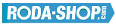# Remote ControlManufacturer Product Image Item Name Price### 608/24-2RS

Miniature Metric Series Chrome Steel Rubber Seals on Both sides Dimension d=8.00 Dimension D=24.00 Dimension B=7.00 Dinamic Load Rating=3293 Static Load Rating=1379 Weight=0.025gr. Speed limit Grease=34.0 Speed limit Oil=40.0
inside diameter = 8.00
outside diameter = 24.00
width = 7.00
€2.24### 608/24-2Z

Miniature Metric Series Chrome Steel Metallic Shields on both sides Dimension d=8.00 Dimension D=24.00 Dimension B=7.00 Dinamic Load Rating=3293 Static Load Rating=1379 Weight=0.025gr. Speed limit Grease=34.0 Speed limit Oil=40.0
inside diameter = 8.00
outside diameter = 24.00
width = 7.00
€2.24### 608/26

Miniature Metric Series Chrome Steel Open(No Shields) Dimension d=8.00 Dimension D=26.00 Dimension B=7.00 Dinamic Load Rating=3293 Static Load Rating=1379 Weight=0.028gr. Speed limit Grease=34.0 Speed limit Oil=40.0
inside diameter = 8.00
outside diameter = 26.00
width = 7.00
€2.24### 608/26-2RS

Miniature Metric Series Chrome Steel Rubber Seals on Both sides Dimension d=8.00 Dimension D=26.00 Dimension B=7.00 Dinamic Load Rating=3293 Static Load Rating=1379 Weight=0.028gr. Speed limit Grease=34.0 Speed limit Oil=40.0
inside diameter = 8.00
outside diameter = 26.00
width = 7.00
€2.24### 608/26-2Z

Miniature Metric Series Chrome Steel Metallic Shields on both sides Dimension d=8.00 Dimension D=26.00 Dimension B=7.00 Dinamic Load Rating=3293 Static Load Rating=1379 Weight=0.028gr. Speed limit Grease=34.0 Speed limit Oil=40.0
inside diameter = 8.00
outside diameter = 26.00
width = 7.00
€2.24### 608W6

Miniature Metric Series Chrome Steel Open(No Shields) Dimension d=8.00 Dimension D=22.00 Dimension B=6.00 Dinamic Load Rating=3293 Static Load Rating=1379 Weight=0.013gr. Speed limit Grease=34.0 Speed limit Oil=40.0
inside diameter = 8.00
outside diameter = 22.00
width = 6.00
€2.24### 609

Miniature Metric Series Chrome Steel Open(No Shields) Dimension d=9.00 Dimension D=24.00 Dimension B=7.00 Dinamic Load Rating=3356 Static Load Rating=1444 Weight=0.016gr. Speed limit Grease=32.0 Speed limit Oil=38.0
inside diameter = 9.00
outside diameter = 24.00
width = 7.00
€2.24### 609-2RS

Miniature Metric Series Chrome Steel Rubber Seals on Both sides Dimension d=9.00 Dimension D=24.00 Dimension B=7.00 Dinamic Load Rating=3356 Static Load Rating=1444 Weight=0.016gr. Speed limit Grease=32.0 Speed limit Oil=38.0
inside diameter = 9.00
outside diameter = 24.00
width = 7.00
€2.24### 609-2Z

Miniature Metric Series Chrome Steel Metallic Shields on both sides Dimension d=9.00 Dimension D=24.00 Dimension B=7.00 Dinamic Load Rating=3356 Static Load Rating=1444 Weight=0.016gr. Speed limit Grease=32.0 Speed limit Oil=38.0
inside diameter = 9.00
outside diameter = 24.00
width = 7.00
€2.24### 61700-2RS

Thin Section Bearings Chrome Steel Rubber Seals on Both sides Dimension d=10.00 Dimension D=15.00 Dimension B=4.00 Dinamic Load Rating=855 Static Load Rating=435 Weight=0.002gr. Speed limit Grease=15.0 Speed limit Oil=17.0
inside diameter = 10.00
outside diameter = 15.00
width = 4.00
€4.24